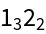#Function Repository Resource:

# WolframModelSignatureForm

Give the formatted form of a signature specification for a Wolfram model rule or state

Contributed by: Stephen Wolfram
 ResourceFunction["WolframModelSignatureForm"][rules] formats the Wolfram model represented by rules in signature form.

## Details and Options

In signature form, a pair like {1,3} is represented as 13.

## Examples

### Basic Examples (1)

Represent a Wolfram model in signature form:

 In:=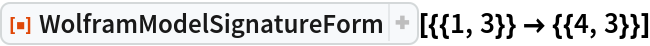Out=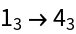### Scope (2)

Convert a Wolfram model rule to signature form:

 In:=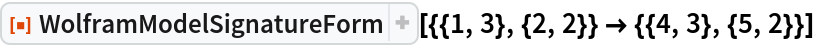Out=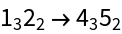Convert a Wolfram model state to signature form:

 In:=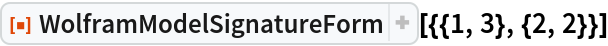Out=